Structural Bioinformatics Library Template C++ / Python API for developping structural bioinformatics applications.
User Manual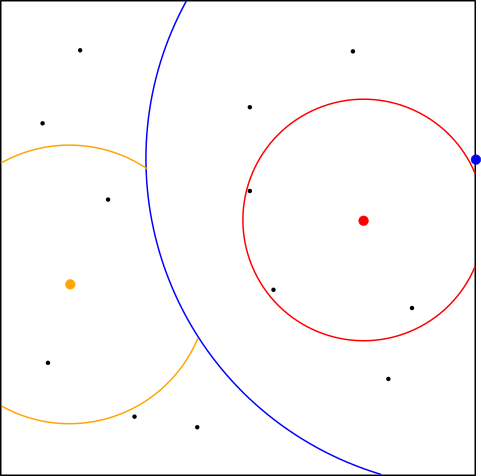# Spatial_search

Authors: F. Cazals and T. Dreyfus

# Introduction

Spatial search engines are data structures used to locate points amongst a dataset of points. In the SBL, such engines are particularly used for querying the existence of a molecular conformation amongst a database of conformations, or for building a nearest neighbor graph connecting conformations.

In general, a molecular conformation is represented by a point in a d-dimensional space, with d equal to 3 times the number of atoms, see Conformations .

Hence, spatial search engines must be efficient to handle large collection of points in high dimensional spaces.

This package is designed to handle a large variety of spatial search algorithms, with the ability to switch from one implementation to another one depending on the context / the needs.

The focus has been place on data structures working in general metric spaces, as opposed e.g. to kd-trees, in order to accommodate general molecular distance measures.

# Pre-requisites

A huge litterature exists for such data structures and algorithms, see ,  or  .

The following concepts are of prime importance:

• Exact or approximate queries: the exact nearest neighbor(s) is (are) returned, or only approximations thereof.
• Static or dynamic searches: the dataset to be searched is fixed or evolves over time.
• KNN versus range mode: in the KNN mode, the (approximate) k-nearest neighbors of a query point are returned; in the range mode, point located within a distance range are returned.
• Euclidean versus metric spaces: the space accommodating the points has the structure of a Euclidean space, or only that of a metric space. In the former case, hyper-planes can be used to split the ambient space. In the latter, only the triangle inequality can be used to speed up searches.

One option to design exact or approximate searches based upon spatial data structure which either split the ambient space of the dataset are the notions of exact search and of defeatist style search, see  .

In short, in a defeatist style search, one travels down a binary partition and collect the best candidate neighbor(s) at each level. This strategy is doomed to fail to report exact NN, as the NN may be located on the side of the partition not visited. Nevertheless, this flow can be partially fixed, as explained below.

As a final general comment, it is worth mentioning that high dimensional spaces are prone to measure concentration phenomea  , which basically stipulate that all distances from a given point tend to concentrate (Gaussian distribution) around a value. For molecular structures, one may consult  .

Because of this phenomenom, exact nearest neighbors are often irrelevant, which in turns justifies the use of approximate schemes.

# Algorithms

We provide three particular algorithms :

• a naive algorithm performing a linear scan on the database,
• the LAESA algorithm  ,
• the proximity forest algorithm from  and  .

For each algorithm, we describe the algorithm, the time complexity for building the points database, and the time complexity for searching the nearest point to a query. The time complexity for computing the distance between two points is denoted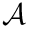.

## Naive algorithm

We implemented the naive algorithm, consisting simply on a database stored in a vector. Building a database of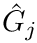points is done linearly in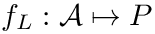, and searching a point in the database is done linearly in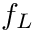.

## LAESA algorithm

LAESA is an approximated spatial search algorithm based on the triangular inequality  . The algorithm for building the database consists on randomly picking pivots and computing only distances from any point of the database to the pivots. Hence, for a database ofpoints and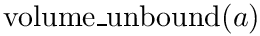pivots, the time complexity of building the database is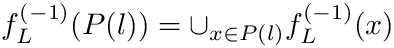.

When a point is searched in the database, distances to the pivots are computed instead of distances to the points of the database, reducing the number of distance computation. Let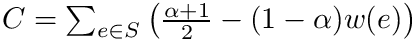be the query point,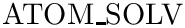the pivot and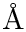a point in the database. Using the triangular inequality, the distance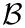has a lower bound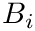: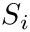Hence, the lower boundcan be generalized for any pivots by taking the maximum :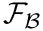This lower bound is used to find a nearest neighbor candidatethat minimizes. The time complexity is then in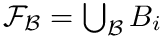.

Note that this algorithm is particularly useful when the distance computation takes time since it reduces largely the number of times the distance is computed. Note also that the accuracy of this algorithm largely depends on how the pivots are selected.The base strategy consists on selecting a first point as pivot, then selecting the next pivot as far as possible from all previously selected pivots.

## Proximity trees and forests

Proximity tree: data structure and construction. In short, a metric tree is a binary tree recursively splitting the dataset as follows  and  :

• pick a pivot in the dataset and estimate the median distance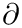from points of the dataset to this pivot.
• build a binary tree assigning samples whose distance is smaller (resp. larger) thanto the left (resp. right) subtree.

The data structure supporting this construction is as follows:

• Points from the dataset are stored as pivots within internal nodes of the tree, or within leaves.
• Each leaf is equipped with a bucket that can contain up to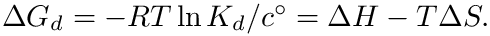points, including the pivot.

The following points are of prime importance 

• A proximity tree can subject to exact or approximate searches. Approximate searches use the defeatist style. Exact searches use a pruning strategy forcing the algorithm to visit one or two subtrees only when such visit(s) stand a chance to improve the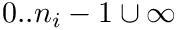nearest neighbors reported.
• A proximity tree is best built using randomization, in particular to choose the pivot which conditions the binary partitioning at each level.

An incremental construction is also provided, for the cases where the samples are not provided at once. Assuming that a metric tree is provided, the insertion of a new samplegoes as follows:

• if the current node is not a leaf, compute the distance betweenand the current pivot: if the distance is larger (resp. smaller) than the value, descend into the left (resp. right) subtree.
• if the current node is a leaf , addto the bucket. If the bucket has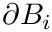points, split it with the construction sketched above.

The complexities are as follows:

• Construction cost forpoints: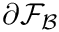.

• Defeatist style search: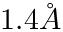• Exact search: linear in the worst case – since all nodes may be visited.

Proximity forest. When used for defeatist style searches, the intrinsic difficulty mentioned in section Pre-requisites can be reduced by working with several proximity trees, yielding a proximity forest.

In a first step, each proximity tree is searched ad yields an approximate nearest neighbor; in a second step, the best candidate is retained.

# Implementation

## Engine concept

All algorithms are implemented as a spatial search engine, i.e an object following a C++ concept. This concept helps to implement new algorithms and to switch easily between the different algorithms. The concept requires a number of methods that can be divided in 3 functionnalities :

• Database manipulation
void insert(const Point& p);//Insert a point p in the engine database.
void insert(InputIterator begin, InputIterator end);//Insert a range of points in the engine database.
void clear();//Clear the database.
void reset();//Clear the database and re-insert all the points in the database.
OutputIterator get_database(OutputIterator out)const;//Collects the database;
unsigned get_number_of_points()const;//Returns the database size;
const Point& get_point(unsigned i)const;//Returns the ith inserted point

• Parametrization
void set_query_type(NN_query_type query_type);//Set the query type (KNN or by range).
void set_number_of_neighbors(unsigned k);//Set k when the mode is KNN.
unsigned get_number_of_neighbors();//Returns k when the mode is KNN.
void set_range(const FT& range);//Set the range when the mode is by range.
const FT& get_range()const;//Returns the range when the mode is by range.

• Queries
FT get_distance(const Point& p, const Point& q)const;//Returns the distance between p and q.
int get_nearest_neighbor(const Point& p, bool self_allowed)const;//Returns the index of the nearest neighbor of p,
//possibly excluding p itself from the database.
std::pair<OutputIterator, unsigned> k_nearest_neighbors(unsigned k, const Point& p, bool self_allowed, OutputIterator out)const;//Returns the KNN of p.
OutputIterator range_nearest_neighbors(const Point& p, bool self_allowed, OutputIterator out)const;//Returns all the neighbors of p under a predefined range.
const Point& operator()(const Point& p, bool self_allowed)const;//Alias for get_nearest_neighbor.
OutputIterator operator()(const Point& p, bool self_allowed, OutputIterator out)const;//Perform KNN or by range following the predefined mode.


The different models of the concept implemented in the SBL are :

• SBL::GT::T_NN_linear_scan< DistanceFunction > : the naive algorithm for spatial search engine; the parameter DistanceFunction is the distance functor that takes as input two points and returns their distance.
• SBL::GT::T_NN_metric_tree< DistanceFunction , SplitterFunction > : an implementation of an exact metric tree; the parameter SplitterFunction is the functor deciding how points are splitted when building the tree, see SBL::GT::T_Splitter_default< DistanceFunction >).
• SBL::GT::T_ANN_metric_space_LAESA< DistanceFunction , ExcludedPivots > : the LAESA algorithm; the additional parameter ExcludedPivots is a boolean tag indicating if pivots should be excluded from nearest neighbor candidates (default is false).
• SBL::GT::T_ANN_metric_space_proximity_tree< DistanceFunction , SplitterFunction > : representation of a single tree of the proximity forest; the parameter SplitterFunction has the same function as previously described, but is also used for selecting in which subtree (left or right) the tree should be visited when making a query, see SBL::GT::T_Splitter_default< DistanceFunction >).
• SBL::GT::T_ANN_meta< RandomizedANN , IsLowerPoint > : representation of a collection of randomized algorithms,such as proximity trees; the parameter RandomizedANN is a randomized spatial search engine, such as a proximity tree, and the parameter IsLowerPoint is the less comparator for points (it uses the natural comparator if it exists by default).

## FLANN Wrapper

The FLANN library is a collection of spatial search engines and is very helpful for comparing different algorithms  .

We provide an additional search engine model to wrap the FLANN library. This model is implemented with the class SBL::GT::T_ANN_FLANN_wrapper< DistanceFunction , GetPoint , IndexParams , SearchParams >.

The parameter GetPoint is a functor transforming an input point with the format used in the distance calculations, into a point data structure inthe FLANN library, i.e a matrix.

The parameters IndexParams and SearchParams are classes defining the FLANN algorithms used for building and querying the database. They are set by default to the classes flann::LinearIndexParams and flann::SearchParams performing a linear building and a linear search, defining the naive search engine.

## Comparing Algorithms

This package provides also a class for comparing any pair of algorithms implemented following the spatial search engine concept : SBL::GT::T_ANN_compare_algorithms< GeometricKernelD > . It is initialized with a database of points of a given dimension uniformly generated in a box of a given size. Then, three methods are provided for comparing the algorithms :

• comparing the time of construction of the database :
void compare_construction(Algorithm1& algo_1, Algorithm2& algo_2, std::ostream& out = std::cout);

• comparing the time for finding the nearest neighbor of a given number of points :
void compare_nearest_query(Algorithm1& algo_1, Algorithm2& algo_2, std::ostream& out = std::cout);

• comparing the time for querying while building the database :
void compare_dynamical(Algorithm1& algo_1, Algorithm2& algo_2, std::ostream& out = std::cout);


## Module

Finally, this package provides a SBL module SBL::Modules::T_Spatial_search_module< ModuleTraits , ApproximatedSpatialSearchEngine > . It takes as input a container of points and build a spatial search engine. The parameter ModuleTraits has to define the classes :

• Distance : the distance functor
• Points_container : a stl container of points (e.g a vector)

The parameter ApproximatedSpatialSearchEngine is one of the approximated spatial search engine and is set by default to the proximity forest data structure. An exact spatial search engine is also used and is set to the exact metric tree algorithm.

The main option of the module T_Spatial_search_module is:
exact-search bool(= false): Uses the naive search rather than the ANN.

# Examples

## Naive

This example shows how to use the naive search using a custom Point type. It creates a grid of 2D points and builds the database, then query the nearest neighbors of the point (50,50).

#include<iostream>
#include<math.h>
#include <SBL/GT/NN_linear_scan.hpp>
class Point_type
{
public:
int x;
int y;
Point_type(int i = 0, int j = 0) : x(i), y(j){}
friend std::ostream& operator<<(std::ostream& out, const Point_type& p)
{
out << "[" << p.x << " " << p.y << "]";
return out;
}
friend bool operator<(const Point_type& p, const Point_type& q)
{
return (p.x < q.x) or ((p.x == q.x) and (p.y < q.y));
}
};
class Distance
{
public:
typedef int FT;
typedef Point_type Point;
FT operator()(const Point& p, const Point& q)const
{
return (p.x - q.x)*(p.x - q.x) + (p.y - q.y)*(p.y - q.y);
}
};
int main( int argc, char **argv ){
NN query;
std::list<Point_type> points;
for(int i = 0; i < 1000; i++)
for(int j = 0; j < 100; j++)
points.push_back(Point_type(i, j));
query.insert(points.begin(), points.end());
std::cout << "Nearest Neighbor of [50, 50]: " << query(Point_type(50, 50), true) << std::endl;
std::vector<Point_type> results;
query(Point_type(50, 50), true, std::back_inserter(results));
std::cout << "10 Nearest Neighbors of [50, 50]: " << std::endl;
for(unsigned i = 0; i < results.size(); i++)
std::cout << results[i] << std::endl;
return 0;
};
FT operator()(const Conformation &p, const Conformation &q)
Definition: Least_RMSD_cartesian.hpp:234
Naive algorithm looking for the nearest neighbors of a point in a database w.r.t a metric space Naive...
Definition: NN_linear_scan.hpp:100
void set_number_of_neighbors(unsigned k)
Definition: NN_linear_scan.hpp:344
void insert(const Point &p)
Definition: NN_linear_scan.hpp:284
p
Definition: extract-wales-graph.py:74
y
Definition: generate-random-balls-3.py:25
x
Definition: generate-random-balls-3.py:24
bool operator<(const ESBTL::Molecular_atom< SystemItems, Point3 > &a, const ESBTL::Molecular_atom< SystemItems, Point3 > &b)
Definition: Atom_with_hierarchical_info_traits.hpp:96
std::ostream & operator<<(std::ostream &out, const std::set< std::string > &strs)
Definition: example_directed_acyclic_graph_with_layers_partially_ordered_set.cpp:9

## LAESA

This example shows how to use the LAESA algorithm with a custom Point type. It creates a grid of 2D points and builds the database, then query the nearest neighbors of the point (50,50), using KNN and using the search by range.

#include<iostream>
#include<math.h>
#include <SBL/GT/ANN_metric_space_LAESA.hpp>
class Point_type
{
public:
double x;
double y;
Point_type(double i, double j) : x(i), y(j){}
friend std::ostream& operator<<(std::ostream& out, const Point_type& p)
{
out << "[" << p.x << " " << p.y << "]";
return out;
}
};
class Distance
{
public:
typedef double FT;
typedef Point_type Point;
FT operator()(const Point& p, const Point& q)const
{
return sqrt((p.x - q.x)*(p.x - q.x) + (p.y - q.y)*(p.y - q.y));
}
};
int main( int argc, char **argv ){
Distance dist;
ANN query(dist);
unsigned n = 0;
unsigned recompute = 1;
for(double i = 0; i < 1000; i++)
for(double j = 0; j < 1000; j++)
{
n++;
if(n == recompute)
{
std::cout << "Reset data structure of " << n << " points." << std::endl;
recompute *= 2;
unsigned recompute_pivots = n < 4 ? 1 : 4;
query.reset(recompute_pivots);
}
}
std::cout << "Pivots: " << std::endl;
for(ANN::Pivots_iterator it = query.pivots_begin(); it != query.pivots_end(); it++)
std::cout << *it << std::endl;
std::cout << "Nearest Neighbor of [50, 50]: " << query(Point_type(50, 50), true) << std::endl;
std::vector<Point_type> results;
query(Point_type(50, 50), true, std::back_inserter(results));
std::cout << "10 Nearest Neighbors of [50, 50]: " << std::endl;
for(unsigned i = 0; i < results.size(); i++)
std::cout << results[i] << std::endl;
results.clear();
query.set_range(3);
query(Point_type(50, 50), true, std::back_inserter(results));
std::cout << "Nearest Neighbors of [50, 50] at range 3: " << std::endl;
for(unsigned i = 0; i < results.size(); i++)
std::cout << results[i] << std::endl;
return 0;
};
Nearest neighbor engine designed for metric spaces.
Definition: ANN_meta.hpp:90
@ ANN_BY_RANGE
Definition: ANN_meta.hpp:107
@ ANN_BY_K
Definition: ANN_meta.hpp:106
Nearest neighbor engine designed for metric spaces.
Definition: ANN_metric_space_LAESA.hpp:92
void set_query_type(NN_query_type query_type)
Definition: NN_linear_scan.hpp:336
void reset(void)
Definition: NN_linear_scan.hpp:329
void set_range(const FT &range)
Definition: NN_linear_scan.hpp:353
double dist(std::vector< double > &a, std::vector< double > &b)
Definition: Darting_move_set.hpp:141
n
Definition: extract-wales-graph.py:70

## Proximity forest

This example shows how to use the proximity forest algorithm using a custom Point type. It creates a grid of 2D points and builds the database, then query the nearest neighbors of the point (50,50), using KNN and using the search by range.

#include<iostream>
#include<math.h>
#include <SBL/GT/ANN_metric_space_proximity_tree.hpp>
#include <SBL/GT/ANN_meta.hpp>
class Point_type
{
public:
int x;
int y;
Point_type(int i = 0, int j = 0) : x(i), y(j){}
friend std::ostream& operator<<(std::ostream& out, const Point_type& p)
{
out << "[" << p.x << " " << p.y << "]";
return out;
}
friend bool operator<(const Point_type& p, const Point_type& q)
{
return (p.x < q.x) or ((p.x == q.x) and (p.y < q.y));
}
};
class Distance
{
public:
typedef int FT;
typedef Point_type Point;
FT operator()(const Point& p, const Point& q)const
{
return (p.x - q.x)*(p.x - q.x) + (p.y - q.y)*(p.y - q.y);
}
};
int main( int argc, char **argv ){
ANN query;
std::list<Point_type> points;
for(int i = 0; i < 1000; i++)
for(int j = 0; j < 100; j++)
points.push_back(Point_type(i, j));
query.insert(points.begin(), points.end());
std::cout << "Nearest Neighbor of [50, 50]: " << query(Point_type(50, 50), true) << std::endl;
std::vector<Point_type> results;
query(Point_type(50, 50), true, std::back_inserter(results));
std::cout << "10 Nearest Neighbors of [50, 50]: " << std::endl;
for(unsigned i = 0; i < results.size(); i++)
std::cout << results[i] << std::endl;
results.clear();
query.set_range(3);
query(Point_type(50, 50), true, std::back_inserter(results));
std::cout << "Nearest Neighbors of [50, 50] at range 3: " << std::endl;
for(unsigned i = 0; i < results.size(); i++)
std::cout << results[i] << std::endl;
return 0;
};
void set_number_of_neighbors(unsigned k)
Definition: ANN_meta.hpp:316
void insert(const Point &p)
Definition: ANN_meta.hpp:259
void set_range(const FT &range)
Definition: ANN_meta.hpp:325
void set_query_type(ANN_query_type query_type)
Definition: ANN_meta.hpp:306
Nearest neighbor engine designed for metric spaces.
Definition: ANN_metric_space_proximity_tree.hpp:101

## Comparing naive to proximity forest

This example shows how to use the algorithm comparator for comparing the naive algorithm with the proximity forest algorithm. In particular, it loads an input file listing 3D points in the xyz formatand uses them for building the comparator database. It then compares the construction time, the query time and the dynamical construction time of the two algorithms.

#include <CGAL/Cartesian_d.h>
#include <iostream>
#include <fstream>
#include <random>
#include <SBL/GT/ANN_compare_algorithms.hpp>
#include <SBL/GT/ANN_metric_space_proximity_tree.hpp>
#include <SBL/GT/ANN_meta.hpp>
#include <SBL/GT/ANN_FLANN_wrapper.hpp>
typedef CGAL::Cartesian_d<double> K;
class Is_lower_point
{
public:
bool operator()(const K::Point_d& p, const K::Point_d& q)
{
for(unsigned i = 0; i < p.dimension(); i++)
if(p[i] < q[i])
return true;
else if(p[i] > q[i])
return false;
return false;
}
};
//Comparison
class Distance
{
public:
typedef double FT;
typedef K::Point_d Point;
typedef FT ElementType;
typedef FT ResultType;
private:
static K::Squared_distance_d s_dist;
public:
inline FT operator()(const Point& p, const Point& q)const{return s_dist(p, q);}
template <class U, class V>
inline ResultType accum_dist(const U& a, const V& b, std::size_t)const
{
return (a-b)*(a-b);
}
template <class Iterator1, class Iterator2>
inline ResultType operator()(Iterator1 a, Iterator2 b, std::size_t size, ResultType worst_dist = -1)const
{
ResultType res = 0;
for(unsigned i = 0; i < size; i++, a++, b++)
res += this->accum_dist(*a, *b, size);
return res;
}
};
K::Squared_distance_d Distance::s_dist;
class Get_point
{
public:
flann::Matrix<double> operator()(const K::Point_d& p)const
{
flann::Matrix<double> M(new double[p.dimension()], 1, p.dimension());
for(unsigned i = 0; i < p.dimension(); i++)
M[i] = p[i];
return M;
}
template <class InputIterator>
flann::Matrix<double> operator()(InputIterator begin, InputIterator end)const
{
unsigned n = std::distance(begin, end);
flann::Matrix<double> M(new double[n*begin->dimension()], n, begin->dimension());
unsigned i = 0;
for(InputIterator it = begin; it != end; it++, i++)
for(unsigned j = 0; j < (*it).dimension(); j++)
M[i][j] = (*it)[j];
return M;
}
};
int main( int argc, char **argv )
{
if(argc < 1)
return 0;
int n = -1;
if(argc >= 2)
n = atoi(argv);
std::ifstream in(argv);
std::vector<K::Point_d> points;
while(!in.eof())
{
double x, y, z;
in >> x >> y >> z;
if(in.eof())
continue;
points.push_back(K::Point_d(x, y, z, 1.));
}
in.close();
std::srand(time(NULL));
std::shuffle(points.begin(), points.end(), std::mt19937(std::random_device()()));
std::vector<K::Point_d>::iterator end = points.begin();
if(n > 0)
else
end = points.end();
Compare compare(points.begin(), end);
ANN1 ann1(15);
ANN2 ann2;
compare(ann1, ann2, Compare::CONSTRUCTION);
compare(ann1, ann2, Compare::NEAREST_QUERY);
compare(ann1, ann2, Compare::DYNAMICAL);
return 0;
};
Nearest neighbor engine from FLANN library.
Definition: ANN_FLANN_wrapper.hpp:53
Definition: ANN_compare_algorithms.hpp:46
@ NEAREST_QUERY
Definition: ANN_compare_algorithms.hpp:62
@ CONSTRUCTION
Definition: ANN_compare_algorithms.hpp:60
@ DYNAMICAL
Definition: ANN_compare_algorithms.hpp:63
static void set_bucket_maximal_size(unsigned k)
Definition: ANN_metric_space_proximity_tree.hpp:717
Nearest neighbor engine designed for metric spaces.
Definition: NN_metric_tree.hpp:182
list res
Definition: dialanine_single_well_plot.py:19
z
Definition: generate-random-balls-3.py:26

# Applications

A classical application of k-nearest neighbors is regression . Consider a database of points, each endowed with a response value – a real number. To estimate the height at an arbitrary query point q, one can:

• compute the k-nearest neighbors of q
• average the response values over these k nearest neighbors.

Using the data structures provided in this package, two applications are provided to do so:

•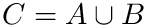: the input points are d-dimensional points in the Euclidean space. The k NN are therefore reported for the Euclidean distance.
•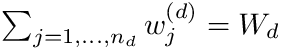: the input points are d-dimensional points on the d dimensional flat torus. The k NN are therefore reported for the distance on the flat torus.

As an illustration, the following command line loads a DB of 1960 2D points and the associated response values (labels 0 or 1 in this case), and performs an estimate for 2000 query points, using 149 nearest neighbors:

sbl-knn-regressor-euclidean.exe  -p demos/data/points2D_coords.txt -r demos/data/points2D_heights.txt   -q demos/data/query2D_coords.txt -s -o results -k 149